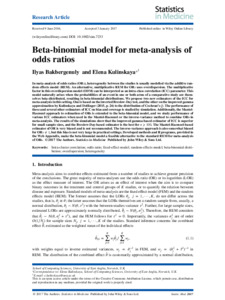# Beta-binomial model for meta-analysis of odds ratios

Bakbergenuly, Ilyas and Kulinskaya, Elena (2017) Beta-binomial model for meta-analysis of odds ratios. Statistics in Medicine, 36 (11). pp. 1715-1734. ISSN 0277-6715Preview PDF (Published manuscript) - Published Version Available under License Creative Commons Attribution. Download (3MB) | Preview

## Abstract

In meta-analysis of odds ratios (\${\OR}s\$), heterogeneity between the studies is usually modelled via the additive random effects model (REM). An alternative, multiplicative random effects model for \${\OR}s\$ uses overdispersion. The multiplicative factor in this overdispersion model (ODM) can be interpreted as an intra-class correlation (ICC) parameter. This model naturally arises when the probabilities of an event in one or both arms of a comparative study are themselves beta-distributed, resulting in beta-binomial distributions. We propose two new estimators of the ICC for meta-analysis in this setting. One is based on the inverted Breslow-Day test, and the other on the improved gamma approximation by Kulinskaya and Dollinger (2015, p. 26) to the distribution of Cochran's \$Q\$. The performance of these and several other estimators of ICC on bias and coverage is studied by simulation. Additionally, the Mantel-Haenszel approach to estimation of odds ratios is extended to the beta-binomial model, and we study performance of various ICC estimators when used in the Mantel-Haenszel or the inverse-variance method to combine odds ratios in meta-analysis. The results of the simulations show that the improved gamma-based estimator of ICC is superior for small sample sizes, and the Breslow-Day-based estimator is the best for \$n\geq100\$. The Mantel-Haenszel-based estimator of \${\OR}\$ is very biased and is not recommended. The inverse-variance approach is also somewhat biased for \${\OR}s\neq1\$, but this bias is not very large in practical settings. Developed methods and R programs, provided in the Web Appendix, make the beta-binomial model a feasible alternative to the standard REM for meta-analysis of odds ratios.

Item Type: Article intra-cluster correlation,odds ratio,fixed-effect model,random-effects model,beta-binomial distribution,overdispersion,heterogeneity Faculty of Science > School of Computing Sciences http://onlinelibrary.wiley.com/doi/10.10... Pure Connector 14 Jan 2017 00:06 12 May 2023 00:27 https://ueaeprints.uea.ac.uk/id/eprint/62085 10.1002/sim.7233View Item